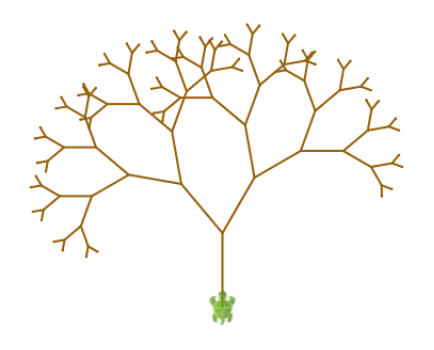## Recursion

This activity has the following desired goals:

• Learning about self-similar structures (A, M).
• Learning to create commands and functions that call themselves (A, M).
• Learning to apply the above ideas to make pleasing drawings (M, T).

### Step 1

Type in the following code and run it:

``````def figure(n: Int) {
forward(n)
right(90)
figure(n - 5)
}
clear()
figure(100)
``````

Q1a. How does the above program work? Trace the program to determine the answer.

Q1b. What is wrong with the program?

### Step 2

Type in the following code and run it:

``````def figure(n: Int) {
if (n < 10) {
forward(n)
}
else {
forward(n)
right(90)
figure(n - 5)
}
}
clear()
figure(100)
``````

Q2a. How does the program in this step improve upon the previous program?

### Step 3

Type in the following code and run it:

``````def numberPattern(n: Int): Int = {
if (n == 1) 2 else numberPattern(n - 1) + 3
}
clearOutput()
repeatFor(1 to 5) { e =>
println(numberPattern(e))
}
``````

Q3a. Does this program use recursion with a command or a function?

Q3b. How does the program calulate the desired numbers? Use tracing to find out.

### Explanation

• A recursive command or function is useful when the solution to a problem depends on the solution to another (e.g. smaller) version of the same problem.
• A recursive command or function calls itself.
• Within a recursive command or function, you deal with a couple of different cases:
• The base case. This handles the version of the problem that does not depend on any another version of the problem. The base case leads to the termination of a recursive solution. Without the base case, a recursive solution will never stop.
• The recursive case. This handles the version of the problem which depends on another (e.g. smaller) version of the problem.

### Exercise

Write a program to make the something like the following figure (notice how the tree is made out of smaller trees; every tree has two smaller trees within it):Contribute Content